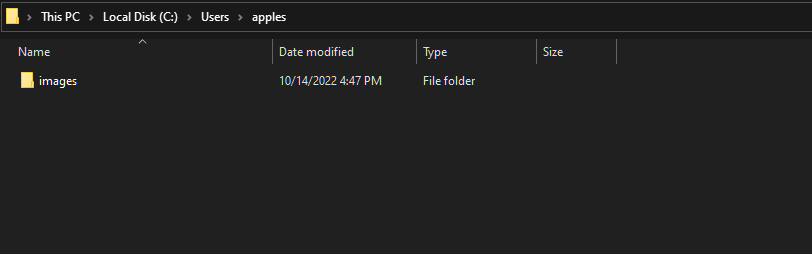GeeksforGeeks App
Open AppBrowser
Continue

# How to Check a File or Directory Exists in C++?

Checking the presence of a directory or a file is one of the most common operations performed by a file system in an Operating System. Most programming languages offer some level of file system accessibility in form of library functions. In this article, you will learn how to test a file or directory that exists in C++.

Note: stat function present in the sys/stat.h header file would be used in the program. The function is not a part of the C++ standard library.

## Checking/Testing the presence of a directory

stat is a pre-defined function present in the sys/stat.h header file. The function takes a path and a structure as arguments in which the metadata associated with the file/directory if present would be stored. The function returns the value of 0 if the path is a valid one. For demonstration we would be testing the presence of the following directory:Example:

## C++

 `// C++ Program to test presence of file/Directory``#include ``#include ``using` `namespace` `std;` `int` `main()``{``    ``// Path to the directory``    ``const` `char``* dir = ``"C:/Users/apples"``;` `    ``// Structure which would store the metadata``    ``struct` `stat sb;` `    ``// Calls the function with path as argument``    ``// If the file/directory exists at the path returns 0``    ``// If block executes if path exists``    ``if` `(stat(dir, &sb) == 0)``        ``cout << ``"The path is valid!"``;``    ``else``        ``cout << ``"The Path is invalid!"``;` `    ``return` `0;``}`

Output:

`The path is valid!`

Explanation: Firstly, the path to the directory is stored in the dir pointer variable. Then the empty structure is initialized with the format that is present in the stat header file. This would store the metadata. Then, inside the if condition where a call to the stat function is made. If the path is valid i.e. the file/directory exists, then the output would be 0, otherwise, it would be non-zero. Hence if the condition would be true if the path is valid, otherwise the else block would be executed, displaying The Path is invalid! message.

## Checking/Testing the presence of a file

The aforementioned process could be used to identify the presence of a file inside a directory as well. With some minor changes to the prior code, the presence of files could also be tested. Therefore, in this example, the attempt to detect the following file would be madeExample:

## C++

 `// C++ Program to test presence of file``#include ``#include ``using` `namespace` `std;` `int` `main()``{``    ``// Path to the directory``    ``const` `char``* file = ``"C:\Temp\4real.png"``;` `    ``// Structure which would store the metadata``    ``struct` `stat sb;` `    ``// Calls the function with path as argument``    ``// If the file/directory exists at the path returns 0``    ``// If block executes if path exists``    ``if` `(stat(file, &sb) == 0 && !(sb.st_mode & S_IFDIR))``        ``cout << ``"The path is valid!"``;``    ``else``        ``cout << ``"The Path is invalid!"``;` `    ``return` `0;``}`

Output:

`The path is valid!`

Explanation: Firstly, the path to the file is stored in the file pointer variable. Then the empty structure is initialized with the format that is present in the stat header file. This would store the metadata. Then, inside the if condition where a call to the stat function is made. If the path is valid i.e. the file exists, then the output would be 0, otherwise, it would be non-zero. Then we check if the path is to a directory using the S_IFDIR flag. If it is then the path is valid but is of a directory, else it is a path to a file.

Note: The process for determining path to a file works on Windows and Linux Distributions.

My Personal Notes arrow_drop_up